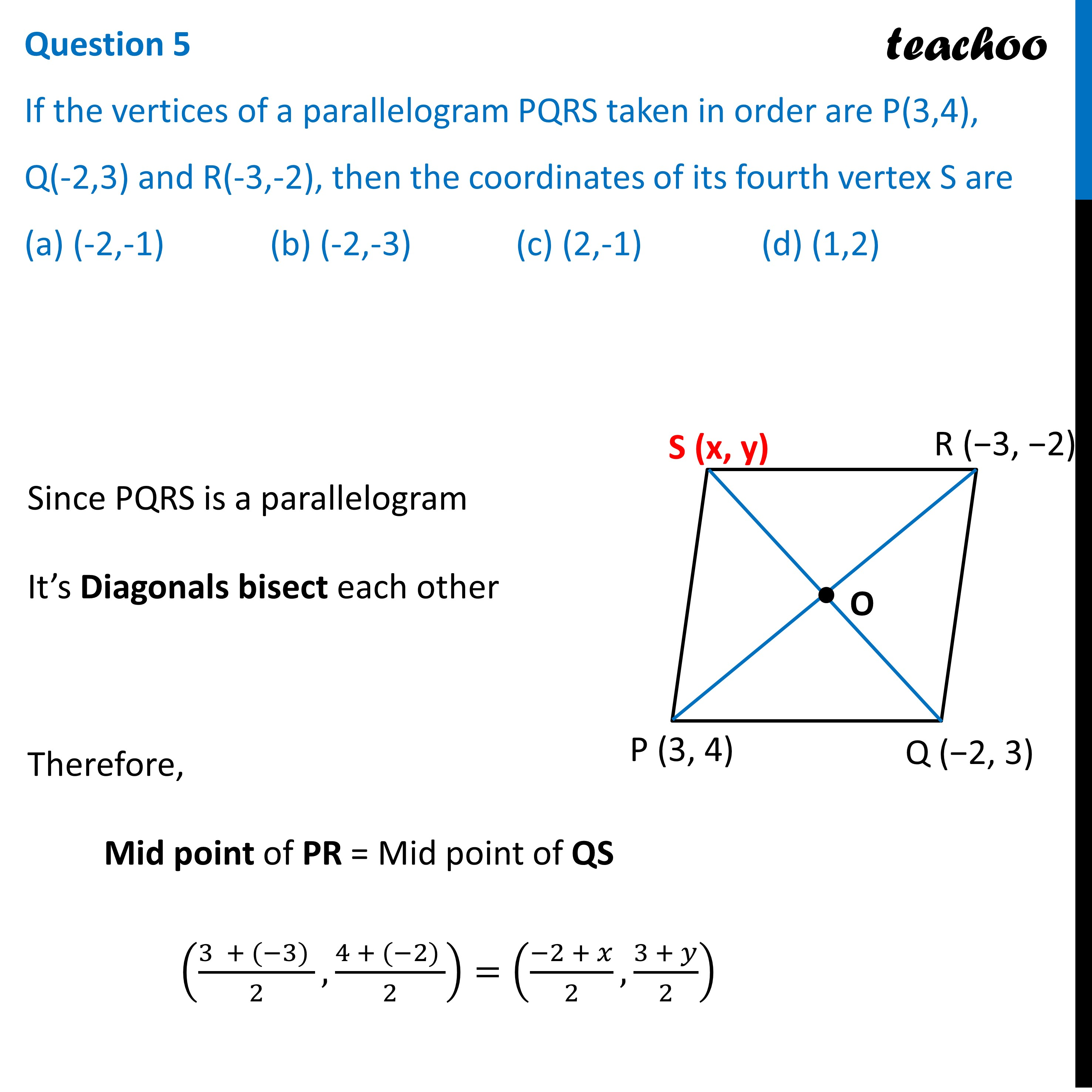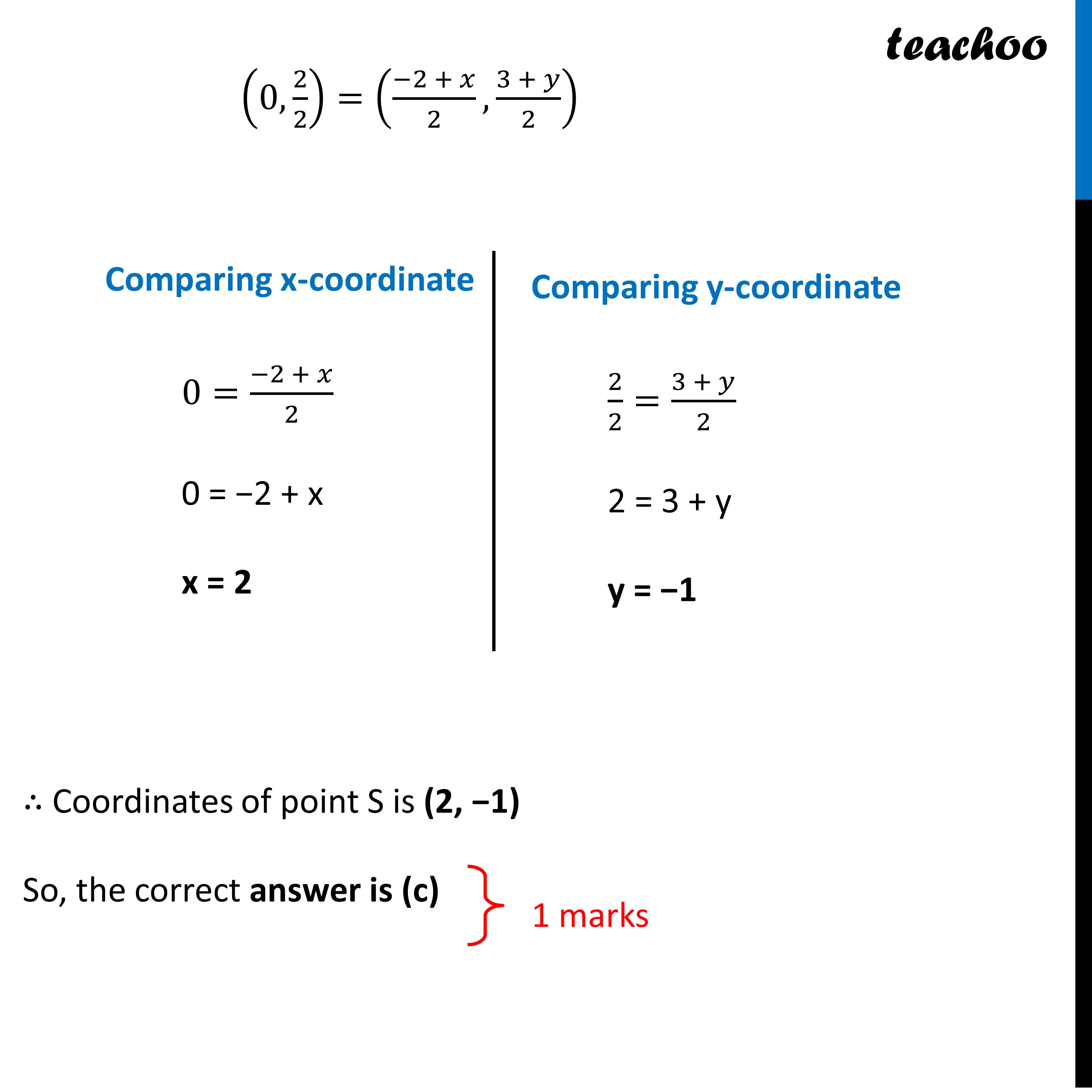CBSE Class 10 Sample Paper for 2023 Boards - Maths Standard

Class 10
Solutions of Sample Papers for Class 10 Boards

## (a) (-2,-1)   (b) (-2,-3)   (c) (2,-1)   (d) (1,2)

This question is similar to Question 14 - NCERT Exemplar - MCQGet live Maths 1-on-1 Classs - Class 6 to 12

### Transcript

Question 5 If the vertices of a parallelogram PQRS taken in order are P(3,4), Q(-2,3) and R(-3,-2), then the coordinates of its fourth vertex S are (a) (-2,-1) (b) (-2,-3) (c) (2,-1) (d) (1,2) Since PQRS is a parallelogram It’s Diagonals bisect each other Therefore, Mid point of PR = Mid point of QS ((3 + (−3) )/2,(4 + (−2) )/2)=((−2 + 𝑥)/2,(3 + 𝑦)/2) (0, 2/2)=((−2 + 𝑥)/2,(3 + 𝑦)/2) (0, 2/2)=((−2 + 𝑥)/2,(3 + 𝑦)/2) Comparing x-coordinate 0=(−2 + 𝑥)/2 0 = −2 + x x = 2 Comparing y-coordinate 2/2=(3 + 𝑦)/2 2 = 3 + y y = −1 ∴ Coordinates of point S is (2, −1) So, the correct answer is (c)